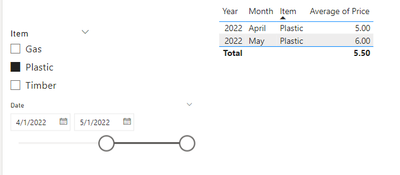cancel
Showing results for
Did you mean:Regular Visitor

## Index 100 based on sliced year and Item

Hi all,

I'm new to PowerBI, so help would be greatly appreciated. Especially since the function 'Index' has come out, the problem should be easier for the experts to solve.

Data

I have the following Table called 'Spend':

 Date Item Price 01/03/2022 Gas 60 01/04/2022 Gas 63 01/05/2022 Gas 64 01/03/2022 Timber 20 01/04/2022 Timber 22 01/05/2022 Timber 26 01/03/2022 Plastic 4 01/04/2022 Plastic 5 01/05/2022 Plastic 6

I want to have a dynamic index model based on the slicers for "date". The index should be 100 (Price / Base Price) with a flexible baseline. For Gas, the index numbers would be 100, 105 and 106,7. However, if the date is changed to April 2022, the index values should become 0, 100, 101.6. Furthermore, it should start again for each unique Item (i.e., Plastic & Timber).

Thank you for helping out!1 ACCEPTED SOLUTIONSuper User

Hi @Justinnn
Please refer to attached sample file with the solution``````Price Index =
VAR BaseTable =
CALCULATETABLE (
TOPN ( 1, 'Table', 'Table'[Date], ASC ),
ALLEXCEPT ( 'Table', 'Table'[Item] ),
ALLSELECTED ( 'Table'[Date] )
)
VAR BasePrice =
MAXX ( BaseTable, 'Table'[Price] )
VAR CurrentPrice =
SELECTEDVALUE ( 'Table'[Price] )
VAR REsult =
DIVIDE ( CurrentPrice, BasePrice ) * 100
RETURN
IF (
NOT ISEMPTY ( CALCULATETABLE ( 'Table', ALL ( 'Table'[Date] ) ) ),
DIVIDE ( CurrentPrice, BasePrice ) * 100 + 0
)``````
2 REPLIES 2Regular Visitor

Hi @tamerj1 ,

Thank you so much for helping me! That was 99% of the code. However, I wanted that the index itself also became flexible. This was my final code for others for future reference.

``````Price Index =
VAR SelectedDate = MINX(ALLSELECTED('Table'[Date]), 'Table'[Date])
VAR BaseTable =
CALCULATETABLE (
TOPN ( 1, 'Table', 'Table'[Date], ASC ),
ALLEXCEPT ( 'Table', 'Table'[Item] ),
'Table'[Date] = SelectedDate
)
VAR BasePrice =
MAXX ( BaseTable, 'Table'[Price] )
VAR CurrentPrice =
SELECTEDVALUE ( 'Table'[Price] )
VAR REsult =
DIVIDE ( CurrentPrice, BasePrice ) * 100
RETURN
IF (
NOT ISEMPTY ( CALCULATETABLE ( 'Table', ALL ( 'Table'[Date] ) ) ),
DIVIDE ( CurrentPrice, BasePrice ) * 100 + 0
) ``````Super User

Hi @Justinnn
Please refer to attached sample file with the solution``````Price Index =
VAR BaseTable =
CALCULATETABLE (
TOPN ( 1, 'Table', 'Table'[Date], ASC ),
ALLEXCEPT ( 'Table', 'Table'[Item] ),
ALLSELECTED ( 'Table'[Date] )
)
VAR BasePrice =
MAXX ( BaseTable, 'Table'[Price] )
VAR CurrentPrice =
SELECTEDVALUE ( 'Table'[Price] )
VAR REsult =
DIVIDE ( CurrentPrice, BasePrice ) * 100
RETURN
IF (
NOT ISEMPTY ( CALCULATETABLE ( 'Table', ALL ( 'Table'[Date] ) ) ),
DIVIDE ( CurrentPrice, BasePrice ) * 100 + 0
)``````